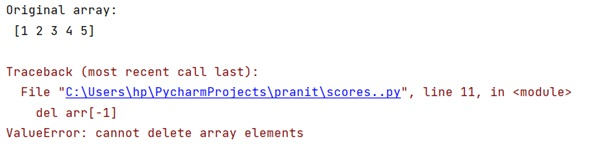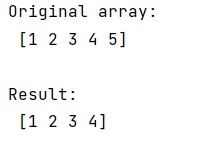# NumPy: What's the best way to remove the last element from 1 dimensional array?

Given a NumPy one dimensional array, we have to remove the last element from it.
Submitted by Pranit Sharma, on March 08, 2023

## Removing the last element from NumPy 1D array

Suppose we are given a 1D array and we need to remove the last element from it.

Since numpy arrays have a fixed size, we cannot remove an element in place. Hence, we cannot use the del keyword.

We can see that the del keyword does not delete the last element of a NumPy array.

### Example

```# Import numpy
import numpy as np

# Creating a numpy array
arr = np.array([1,2,3,4,5])

# Display original array
print("Original array:\n",arr,"\n")

# Deleting last element
del arr[-1]

# Display result
print("Result:\n",arr,"\n")
```

### OutputTo remove the last element from 1 dimensional array, we need to create a new view of the array containing all elements except the last one using the slice notation.

However, a view shares the data with the original array, so if one is modified so is the other.

Let us understand with the help of an example,

## Python code to remove the last element from 1 dimensional array

```# Import numpy
import numpy as np

# Creating a numpy array
arr = np.array([1,2,3,4,5])

# Display original array
print("Original array:\n",arr,"\n")

# Deleting last element
res = arr[:-1]
arr = res

# Display result
print("Result:\n",arr,"\n")
```

### OutputLanguages: » C » C++ » C++ STL » Java » Data Structure » C#.Net » Android » Kotlin » SQL
Web Technologies: » PHP » Python » JavaScript » CSS » Ajax » Node.js » Web programming/HTML
Solved programs: » C » C++ » DS » Java » C#
Aptitude que. & ans.: » C » C++ » Java » DBMS
Interview que. & ans.: » C » Embedded C » Java » SEO » HR
CS Subjects: » CS Basics » O.S. » Networks » DBMS » Embedded Systems » Cloud Computing
» Machine learning » CS Organizations » Linux » DOS
More: » Articles » Puzzles » News/Updates